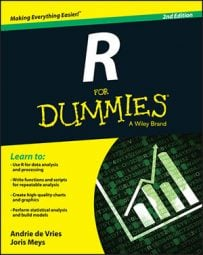##### R For DummiesWhenever you have a limited number of different values in R, you can get a quick summary of the data by calculating a frequency table. A frequency table is a table that represents the number of occurrences of every unique value in the variable. In R, you use the table() function for that.

## Creating a table in R

You can tabulate, for example, the amount of cars with a manual and an automatic gearbox using the following command:

```> amtable <- table(cars\$am)
> amtable
auto manual
13   19```

This outcome tells you that your data contains 13 cars with an automatic gearbox and 19 with a manual gearbox.

## Working with tables in R

As with most functions, you can save the output of table() in a new object (in this case, called amtable). At first sight, the output of table() looks like a named vector, but is it?

```> class(amtable)
 "table"```

The table() function generates an object of the class table. These objects have the same structure as an array. Arrays can have an arbitrary number of dimensions and dimension names. Tables can be treated as arrays to select values or dimension names.